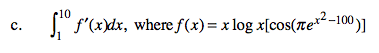### Home > CALC > Chapter 6 > Lesson 6.5.1 > Problem6-173

6-173.
1. Simplify each expression. Homework Help ✎

1.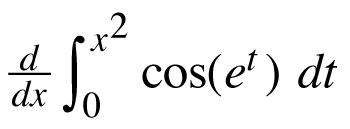2.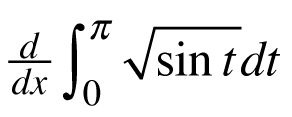3.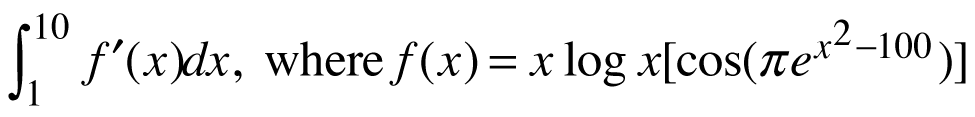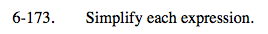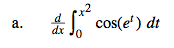Fundamental Theorem of Calculus states that the derivative of an INDEFINITE integral gives you the original function.
But this is a definite integral...

Be sure to multiply by the derivative of the bounds.

(2x)cos (ex2) − (0)cos(e0) = 2xcosex2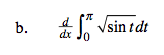Refer to the hints in part (a), or... Notice that the definite integral equals a constant. What is the derivative of a constant?